# Common Core: 7th Grade Math : Divide Integers: CCSS.Math.Content.7.NS.A.2b

## Example Questions

← Previous 1 3 4 5 6 7

### Example Question #1 : Divide Integers: Ccss.Math.Content.7.Ns.A.2b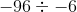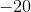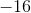Explanation:

When two negative numbers are divided, the answer is positive. Divide normaly. Answer is.

### Example Question #2 : Divide Integers: Ccss.Math.Content.7.Ns.A.2b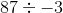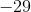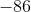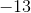Explanation:

When a positive number and a negative number is divided, the answer is negative. Just divide normally. Answer is.

### Example Question #3 : Divide Integers: Ccss.Math.Content.7.Ns.A.2b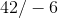Explanation:

When dividing a negative number and a positive number, our answer is negative. Divide normally.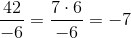Answer is.

### Example Question #1 : Divide Integers: Ccss.Math.Content.7.Ns.A.2b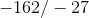Explanation:

When dividing two negative numbers, our answer is positive. Divide normally.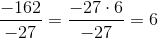Answer is.

### Example Question #1 : Divide Integers: Ccss.Math.Content.7.Ns.A.2b

Solve: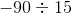Explanation:

When dividing a negative number and a positive number, our answer is negative. Divide normally. Answer is.

### Example Question #1 : Divide Integers: Ccss.Math.Content.7.Ns.A.2b

Solve: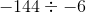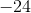Explanation:

When dividing two negative numbers, the answer is positive. Then divide normally. Answer is.

### Example Question #7 : Divide Integers: Ccss.Math.Content.7.Ns.A.2b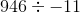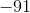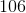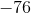Explanation:When dividing with a negative number, our answer is negative.

Answer is.

### Example Question #1 : Divide Integers: Ccss.Math.Content.7.Ns.A.2b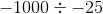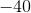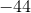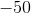Explanation:When dividing with two negative numbers, our answer is positive.

Answer is### Example Question #91 : Negative Numbers

Solve: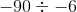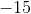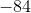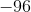Explanation:

When dividing with two negative values, the answer becomes positive. Then we just divideby, which equals. The answer is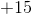or### Example Question #10 : Divide Integers: Ccss.Math.Content.7.Ns.A.2b

Solve: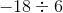When dividing with negative numbers, we count the number of negative numbers. We have one so that means the answer is negative. So the quotient is